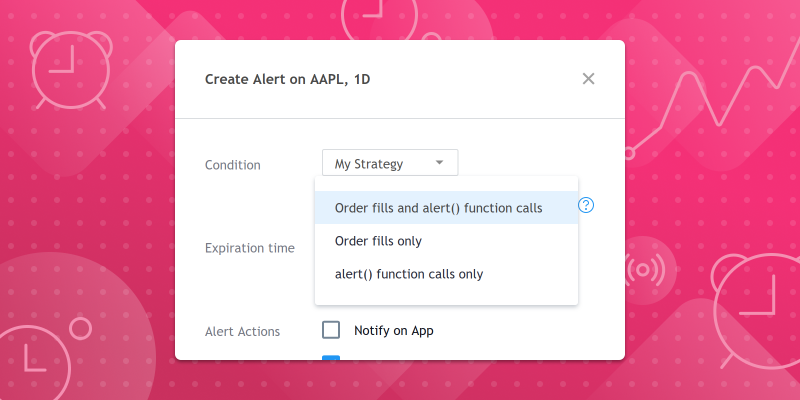# Our new alerts allow for dynamic messages

Jan 25 2021Our new script alerts use an`alert()` function which works in both strategies and studies, and allows a fully dynamic message to be generated when the alert triggers.

The new alerts work using a model similar to the recent strategy alerts, where only one alert created in the chart user interface can aggregate all the triggers generated by any number of `alert()` calls in the script, in the same way that a single strategy alert would aggregate all broker event triggers.

1. Include as many `alert()` calls in your script as you wish, enclosing each one in an `if` block defining the triggering condition(s).
2. Create one alert for the script using the chart’s “Create Alert” dialog box and select an alert type including “alert() function call”.

When used in strategies, users can choose to create alerts that trigger only on `alert()` events, on order fill events, or on both. Also it’s worth remembering that there’s no limit on the number of variables that can be used in the dynamic alert message and that placeholders are no longer necessary, as any variable used in a script can also be used in the new `alert()` function calls, as long as it is in string format.

Below are three examples of the `alert()` function:

1) The script alert is triggered on each bar, and the message contains the bar’s closing price:

```//@version=4
plot(close)

2) The values of three indicators are checked: RSI, SMA and Momentum. The script alert will be triggered if any of the indicators crosses a specified level (or price), and the message will contain the name of the indicator and its current value.

```//@version=4
f_triggerSma()=>
_s  = sma(close, 14)
_co = crossover(close, _s)
_cu = crossunder(close, _s)
if _co
alert("Price (" + tostring(close) + ") crossing up SMA (" + tostring(_s) + ")", alert.freq_once_per_bar)
else if _cu
alert("Price (" + tostring(close) + ") crossing down SMA (" + tostring(_s) + ")", alert.freq_once_per_bar)
f_triggerRsi()=>
_r  = rsi(close, 7)
_co = crossover(_r, 70)
_cu = crossunder(_r, 30)
if _co
else if _cu
f_triggerMom()=>
_m  = mom(close, 14)
_co = crossover(_m, 0)
_cu = crossunder(_m, 0)
if _co
else if _cu

plot(sma(close, 14), "SMA")
f_triggerSma()
f_triggerRsi()
f_triggerMom()```

3) In this example the values of one RSI indicator are checked, but on five symbols at once. The script alert will be triggered if RSI crosses a specified level for any of the symbols, and the message will contain the symbol and the indicator value at the moment of crossing.

```//@version=4
f_triggerRsi(_ticker)=>
_r = rsi(close, 7)
_x = crossover(_r,70)
_y = crossunder(_r,30)
_rt = barstate.isrealtime
[_rsi, _co, _cu, _rt_bar] = security(_ticker, timeframe.period, [_r, _x, _y, _rt])
_msg = _ticker + ", " + timeframe.period + ": "
if _co and _rt_bar
_msg := _msg + "RSI (" + tostring(_rsi) + ") crossing up 70 level"
else if _cu and _rt_bar
_msg := _msg + "RSI (" + tostring(_rsi) + ")  crossing down 30 level"

plot(rsi(close, 7), "RSI", color=#8E1599)
band1 = hline(70, "Upper Band", color=#C0C0C0)
band0 = hline(30, "Lower Band", color=#C0C0C0)
fill(band1, band0, color=color.new(#9915FF,90), title="Background")

f_triggerRsi(syminfo.tickerid)
f_triggerRsi("NASDAQ:MSFT")
f_triggerRsi("FX:EURUSD")
f_triggerRsi("NASDAQ:TSLA")
f_triggerRsi("NASDAQ:PYPL")```

This script shows how you can use `alert()` in a strategy, to generate alert messages containing orders for a third-party execution engine.